# How to remember the standard deviation

The standard deviation formula is:

Standard deviation =sigma=sqrt((Sigma(x-bar x)^2)/(n-1))

1.  Find the mean (of all the numbers) = barx

2.  Subtract the mean (from each number) = x-barx

3.  Square the result (of each of the above) = (x-barx)^2

4.  Add the results up (Add) =sum(x-barx)^2

5.  Divide (the result) by the number of data values minus one =(sum(x-barx)^2)/(n-1)

6.  Take the square root of the result =sqrt((sum(x-barx)^2)/(n-1))

 To set the standards Standard Any deviants Deviation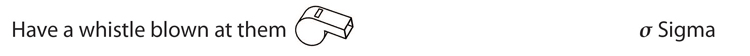Standard deviation =sigma

and these mean ones are taken out of the class =x-barx

Each\ \n\u\m\b\er - mean

they are all put in the school square =s\q\u\a\r\e

(Each\ \n\u\m\b\er - mean)^2

then there is a roll call to add up the deviants =ADD

sum(Each\ \n\u\m\b\er - mean)^2

then they have to run over ninety miles =\ \/n

(sum(Each\ \n\u\m\b\er - mean)^2)/n

there is always one that doesn't make it back =-1

(sum(Each\ \n\u\m\b\er - mean)^2)/(n-1)

This roots out any problems

sqrt((sum(Each\ \n\u\m\b\er - mean)^2)/(n-1))

So

Standard deviation =sigma=sqrt((sum(x-barx)^2)/(n-1))

In more detail and with pictures you would remember this as follows: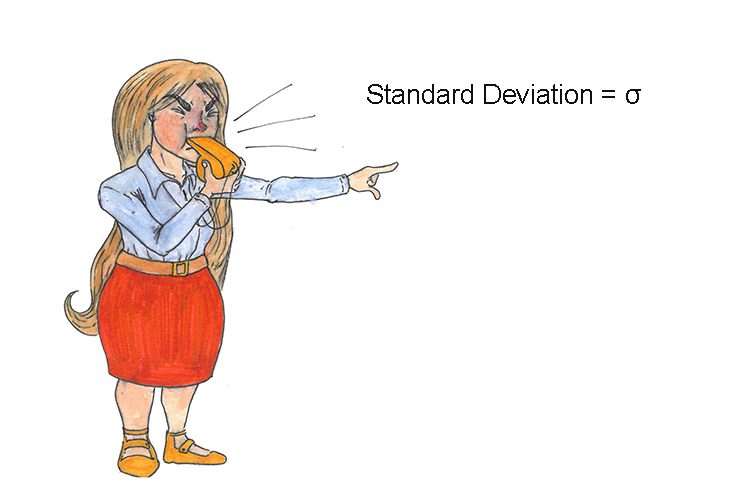To set the standards any deviants have a whistle blown at them.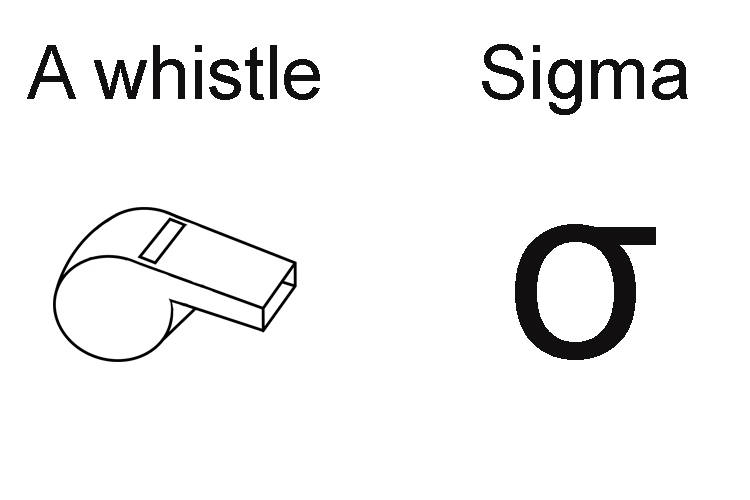A whistle and sigma look very alike.

Subtract the mean.

Then square each result.All these mean ones are then marched into the school square.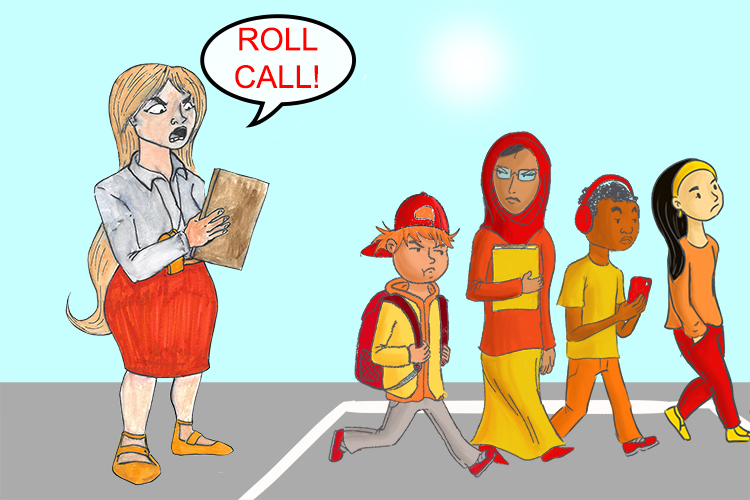There is a roll call to add up all the deviants.

NOTE:

If you just learnt up to this point this would help you enormously with how to work out what the equation means.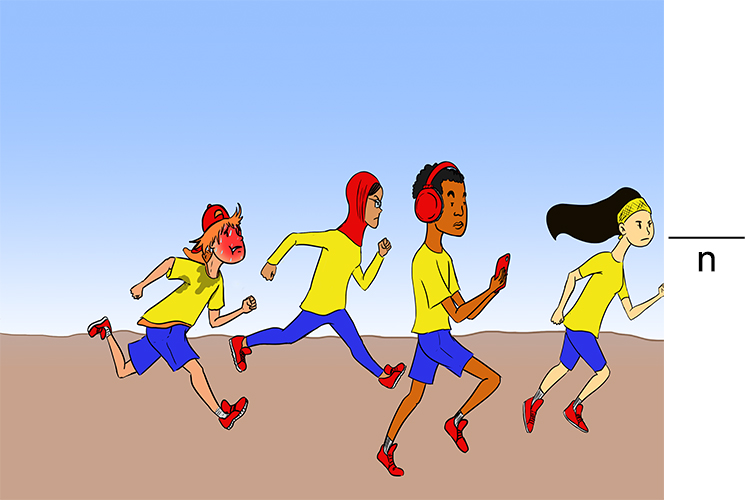The deviants are made to run over (÷) ninety (n) miles.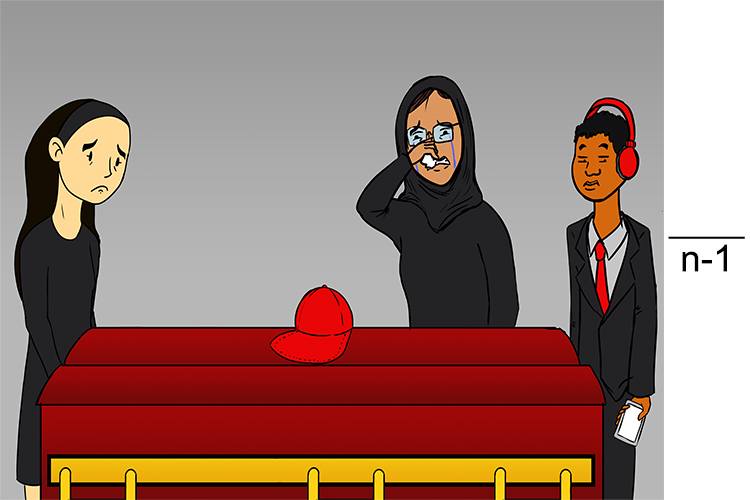There is always one that doesn’t make it back (-1).

Square root the result.This roots out any problems (√).